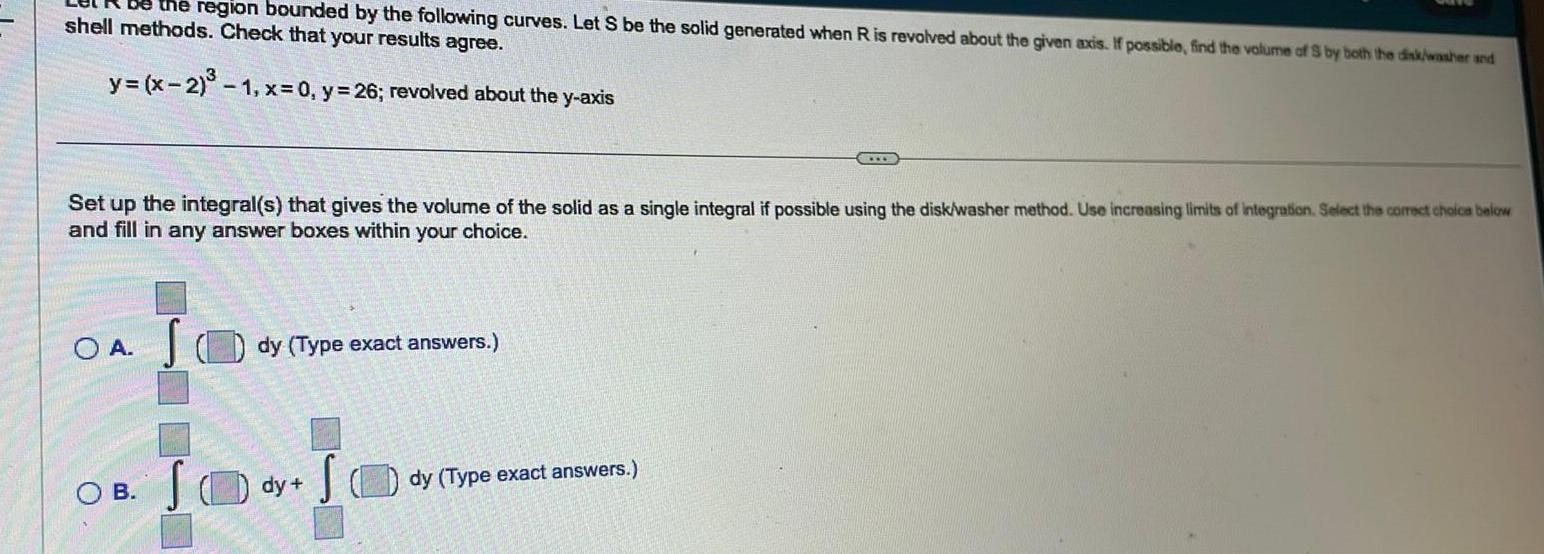Question:

# be the region bounded by the following curves Let S be the

Last updated: 11/19/2023be the region bounded by the following curves Let S be the solid generated when R is revolved about the given axis If possible find the volume of S by both the disk washer and shell methods Check that your results agree y x 2 1 x 0 y 26 revolved about the y axis Set up the integral s that gives the volume of the solid as a single integral if possible using the disk washer method Use increasing limits of integration Select the correct choice below and fill in any answer boxes within your choice OA S OB dy Type exact answers S SO dy dy Type exact answers